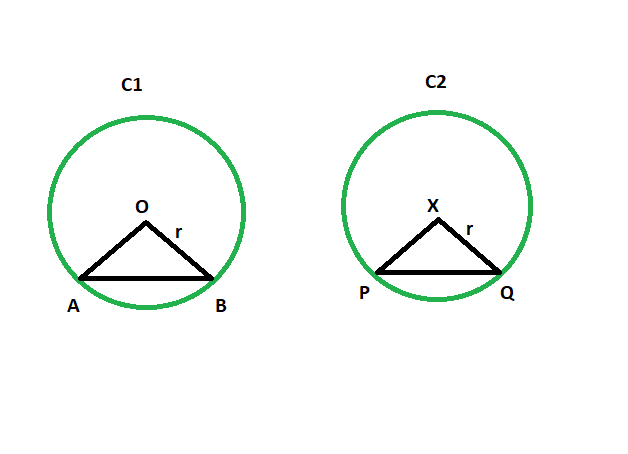# Angle subtended by the chord to center of the circle when the angle subtended by the another equal chord of a congruent circle is given

Given are two congruent circles with two equal chords. The angle subtended to the centre from the chord of one of the circles is given. The task is to find the angle subtended by the chord to the centre of another circle.

Examples:

```Input: z = 48
Output: 48 degrees

Input: z = 93
Output: 93 degrees
```Approach:

• In triangle AOB and PXQ
```AO = PX(radii of congruent circles)
BO = QX(radii of congruent circles)
AB = PQ(equal chords)
```
• So, triangle AOB is congruent with triangle PXQ
• So, angle AOB = angle PXQ

Equal chords of congruent circles subtend equal angles at their centres.

Below is the implementation of the above approach:

## C++

 `// C++ program to find the angle subtended by the chord ` `// to the centre of the circle when the angle subtended ` `// by another equal chord of a congruent circle is given ` ` `  `#include ` `using` `namespace` `std; ` ` `  `void` `anglequichord(``int` `z) ` `{ ` `    ``cout << ``"The angle is "` `<< z ` `         ``<< ``" degrees"` `<< endl; ` `} ` ` `  `// Driver code ` `int` `main() ` `{ ` `    ``int` `z = 48; ` `    ``anglequichord(z); ` `    ``return` `0; ` `} `

## Java

 `// Java program to find the angle subtended by the chord ` `// to the centre of the circle when the angle subtended ` `// by another equal chord of a congruent circle is given ` ` `  `import` `java.io.*; ` ` `  `class` `GFG  ` `{ ` `     `  `static` `void` `anglequichord(``int` `z) ` `{  ` `    ``System.out.println (``"The angle is "` `+ z + ``" degrees"``); ` `} ` ` `  `// Driver code ` `public` `static` `void` `main (String[] args)  ` `{ ` ` `  `    ``int` `z = ``48``; ` `    ``anglequichord(z); ` ` `  `} ` `} `

## Python 3

 `# Python 3 program to find the angle subtended by the chord ` `# to the centre of the circle when the angle subtended ` `# by another equal chord of a congruent circle is given ` ` `  ` `  `def` `anglequichord(z): ` `    ``print` `(``"The angle is "` `, z ` `        ``, ``" degrees"``) ` ` `  `# Driver code ` `if` `__name__ ``=``=` `"__main__"``: ` `    ``z ``=` `48` `    ``anglequichord(z) ` ` `  `# This code is contributed by ChitraNayal `

## C#

 `// C# program to find the angle subtended by the chord  ` `// to the centre of the circle when the angle subtended  ` `// by another equal chord of a congruent circle is given  ` `using` `System; ` ` `  `class` `GFG  ` `{  ` `     `  `    ``static` `void` `anglequichord(``int` `z)  ` `    ``{  ` `        ``Console.WriteLine(``"The angle is "` `+ z + ``" degrees"``);  ` `    ``}  ` `     `  `    ``// Driver code  ` `    ``public` `static` `void` `Main ()  ` `    ``{  ` `     `  `        ``int` `z = 48;  ` `        ``anglequichord(z);  ` `    ``}  ` `}  ` ` `  `// This code is contributed by AnkitRai01 `

Output:

```The angle is 48 degrees
```

My Personal Notes arrow_drop_upCheck out this Author's contributed articles.

If you like GeeksforGeeks and would like to contribute, you can also write an article using contribute.geeksforgeeks.org or mail your article to contribute@geeksforgeeks.org. See your article appearing on the GeeksforGeeks main page and help other Geeks.

Please Improve this article if you find anything incorrect by clicking on the "Improve Article" button below.

Improved By : jit_t, AnkitRai01, chitranayal

Article Tags :
Practice Tags :

Be the First to upvote.

Please write to us at contribute@geeksforgeeks.org to report any issue with the above content.